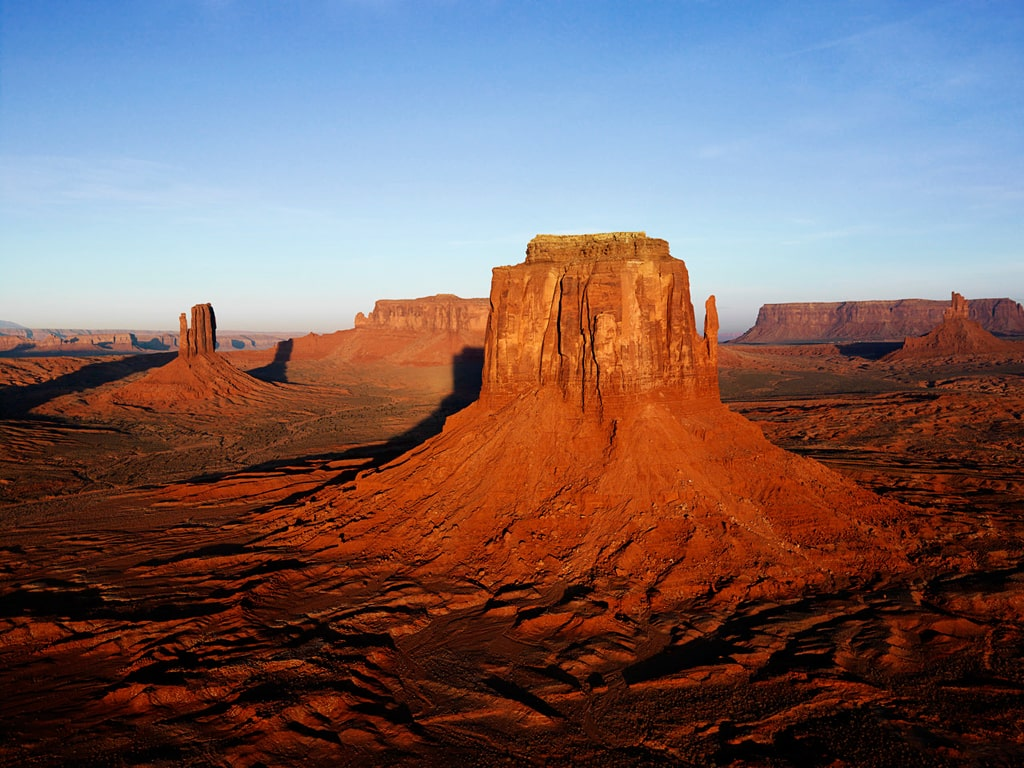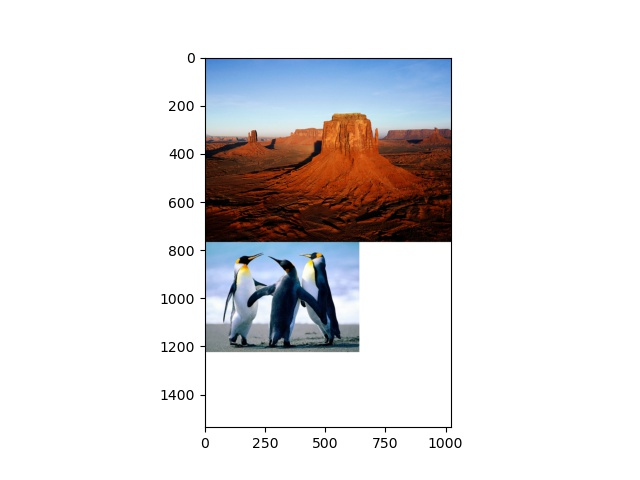### How to Add Two Images of Different Size Vertically and Horizontally in OpenCV Python?

In this post, we learn how to add two images of different size vertically and horizontally using OpenCV Python. We use OpenCV Python methods such as cv2.vconcat()  and cv2.hconcat() to add images. These methods can add images with same size only. So to make the sizes the same, we add borders (with black or white pixels) to images using cv2.copyMakeBorder().

We will cover the contents listed in the following steps.

#### Steps to add images of different size vertically and horizontally:

We will be using the following two images as the inputs to the programs discussed in this post.Desert.jpgPenguins.jpg

Prerequisites
• Python 3
• OpenCV
• NumPy
• Matplotlib (Optional)

## STEP 1: Import Required Libraries/ Packages

The first step of any python programming is importing the required packages and libraries. We import the OpenCV (cv2) and Matplotlib. We used Matplotlib to display the images showing width and height. Using Matplotlib is optional.
`import cv2import matplotlib.pyplot as plt`

## STEP 2: Load the Images

The next step is to load the images. We load to images "Desert.jpg" and "Penguins.jpg". You can download the images and follow the process with the same images

Here you should change the image path accordingly.
`# Load the input images img1 = cv2.imread('Desert.jpg')img2 = cv2.imread('Penguins.jpg')`
We have discussed different ways to load image in Python in our previous post.

### STEP 3: Get height and width of images

First find the height and width of the images.
`# Get height and width of first imageheight1, width1 = img1.shape,img1.shapeprint(height1, width1)`
Output
768  1024

`# Get height and width of the second imageheight2, width2 = img2.shape,img2.shapeprint(height2, width2)`
Output
457  640

Notice the two images have different sizes (height and width).

Now we move to add images (of different size) vertically and horizontally.

### STEP 4: Find max height and width of images

Here we find the max height and width.
`# Find Max height and width of imagesmax_height = max(height1, height2)max_width = max(width1, width2)`

### STEP 5: Add borders to the images to have same width and height using copyMakeBorder() method

To make the size of image the same we add border to the images using copyMakeBorder() method. We filled with ones in the border area. You can fill with zeros.
Have a look at the below code snippet.
`# Add borders the images to have same width and #height using copyMakeBorder() methodimg1 = cv2.copyMakeBorder(src = img1,                          top = 0,                          bottom=max_height-height1,                           left=0,                           right =max_width-width1,                           borderType = cv2.BORDER_CONSTANT,                           value=[255,255, 255]                          )`
`img2 = cv2.copyMakeBorder(src = img2,                           top = 0,                           bottom=max_height-height2,                           left=0,                           right =max_width-width2,                           borderType = cv2.BORDER_CONSTANT,                           value=[255,255, 255]                          )`
Here we get resized images with same size (max height and width).
We can add borders to top, bottom, left and right of images. We add border to bottom and right of the images.
Note: You can set value to zero as value = [0,0,0] to make border area black.

### STEP 6: Add resized images vertically and horizontally

Now we add these resized images vertically or horizontally.
`# Add resized images vertically and horizontallyimg_add_v = cv2.vconcat([img1,img2]) img_add_h = cv2.hconcat([img1,img2])`

### STEP 7: Display the vertically and horizontally added images

Let's visualize the vertically (or horizontally) added images.
`#Display vertically added imagescv2.imshow('added image',img_add_v)cv2.waitKey(0)cv2.destroyAllWindows()`
`#Display horizontally added imagescv2.imshow('added image',img_add_v)cv2.waitKey(0)cv2.destroyAllWindows()`

Now to visualize using Matplotlib we need convert the images from BGR to RGB.
Note-If you have already convert the original images from BGR to RGB format, no need to again convert. So skip the below step.
`# Convert the image from BGR to RGBimg_add_h = cv2.cvtColor(img_add_h, cv2.COLOR_BGR2RGB)img_add_v = cv2.cvtColor(img_add_v, cv2.COLOR_BGR2RGB)`
Now visualize the vertically (or horizontally added) images.
`# Display vertically added imagesplt.imshow(img_add_v)plt.show()`

OutputVertically added images

Now visualize the horizontally added images.
`# Display horizontally added imagesplt.imshow(img_add_h)plt.show()`

OutputHorizontally Added Images

Wow!!! we have accomplished all our task successfully. We have added images (of different sizes) vertically and horizontally.

## Complete Program 3 (add images of different sizes vertically and horizontally)

Now look at the complete program to add images of different sizes vertically and horizontally.
`# %matplotlib qt# impolrt required librariesimport cv2import matplotlib.pyplot as plt# Read two imagesimg1 = cv2.imread('Desert.jpg')img2 = cv2.imread('Penguins.jpg')# Find height and width of the imagesheight1, width1 = img1.shape,img1.shapeheight2, width2 = img2.shape,img2.shape# Find Max height and width of imagesmax_height = max(height1, height2)max_width = max(width1, width2)# Add borders the images to have same width and#height using copyMakeBorder() methodimg1 = cv2.copyMakeBorder(src = img1,top = 0,bottom=max_height-height1,left=0,right =max_width-width1,borderType = cv2.BORDER_CONSTANT,value=[255,255, 255])img2 = cv2.copyMakeBorder(src = img2,top = 0,bottom=max_height-height2,left=0,right =max_width-width2,borderType = cv2.BORDER_CONSTANT,value=[255,255, 255])# Add resized images vertically and horizontallyimg_add_v = cv2.vconcat([img1,img2])img_add_h = cv2.hconcat([img1,img2])# Display vertically and horizontally added imagescv2.imshow('added image',img_add_v)cv2.waitKey(0)cv2.destroyAllWindows()# Display horizontally added images#cv2.imshow('added image',img_add_h)#cv2.waitKey(0)#cv2.destroyAllWindows()# Convert the image from BGR to RGBimg_add_h = cv2.cvtColor(img_add_h, cv2.COLOR_BGR2RGB)img_add_v = cv2.cvtColor(img_add_v, cv2.COLOR_BGR2RGB)# Display vertically dded imagesplt.imshow(img_add_v)plt.show()# Display horizontally added images#plt.imshow(img_add_h)#plt.show()`

We have covered all the content related to adding two images (of different size) vertically as well as horizontally. If you have any doubts or want more related to this topic please comment.